Published

# PDDU(Portable Distance Display Unit)

The PDDU uses HCSR04 sonar sensor to measure distance and print it on an 16:2 lcd screen. Using a battery makes the device portable.

BeginnerFull instructions provided59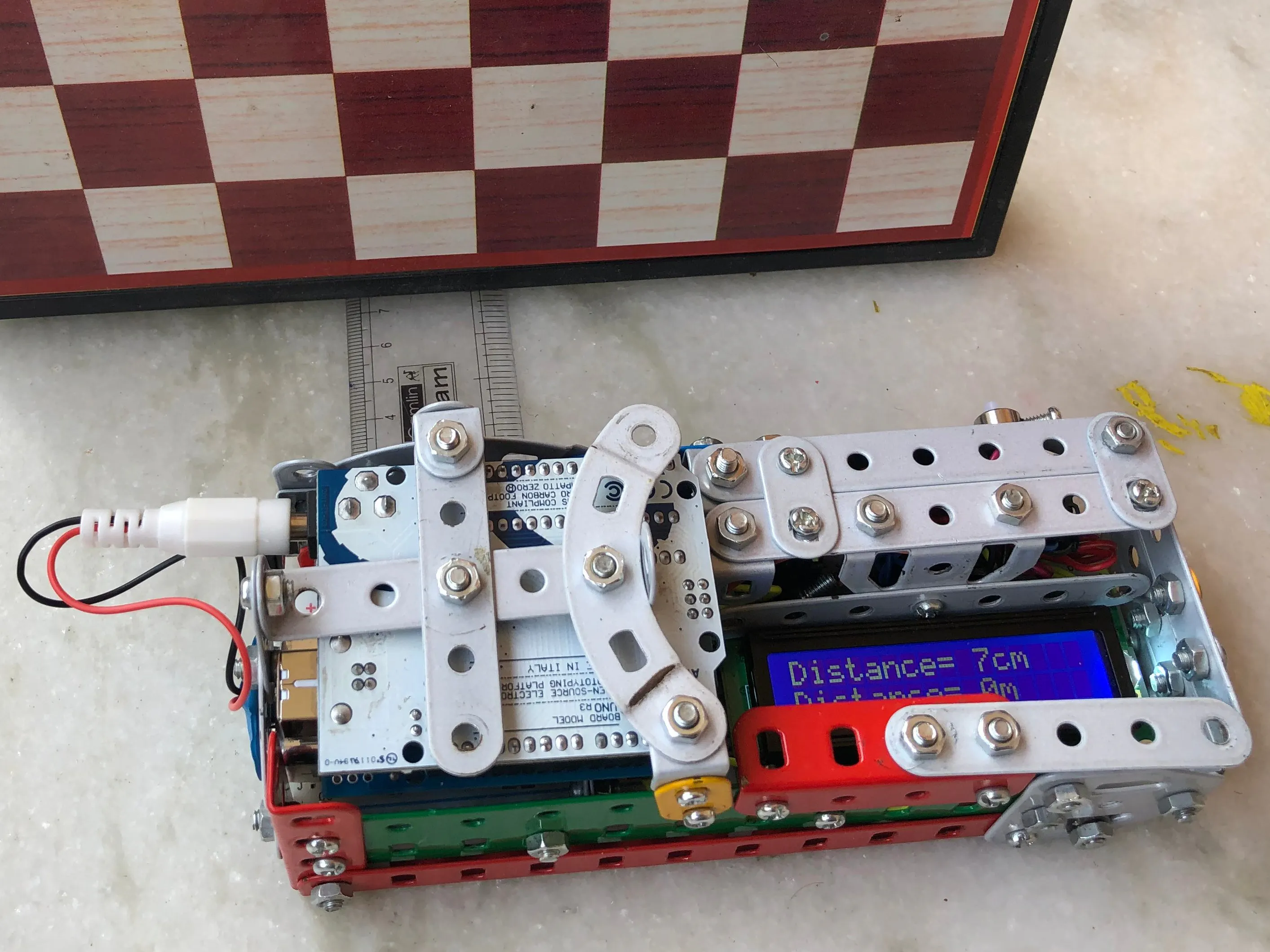## Things used in this project

### Hardware components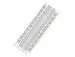×1Arduino UNO & Genuino UNO
×1Ultrasonic Sensor - HC-SR04 (Generic)
×1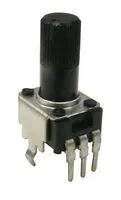Rotary Potentiometer, 10 kohm
×1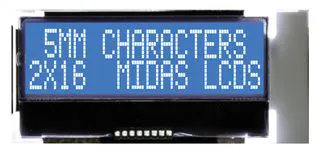Alphanumeric LCD, 16 x 2
×1Jumper wires (generic)
×1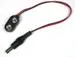9V to Barrel Jack Connector
×1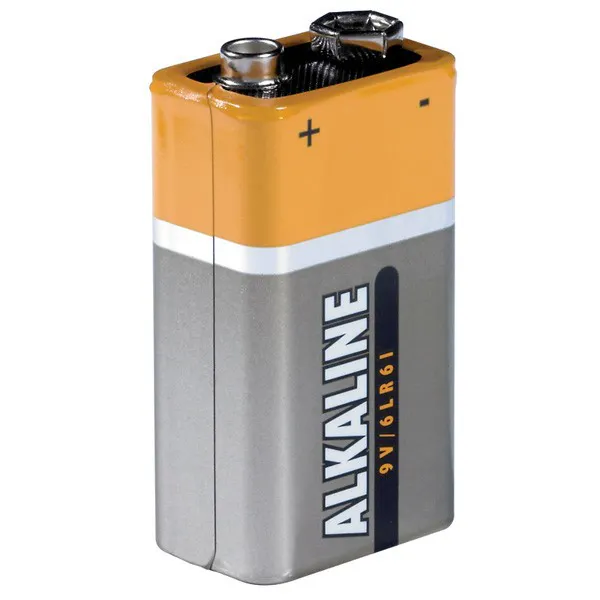9V battery (generic)
×1

### Software apps and online services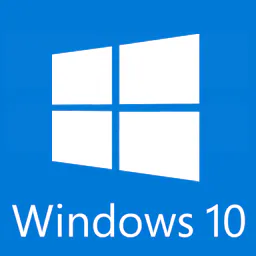Microsoft Windows 10Arduino IDE

## Schematics

### PDDU circuit diagram and schematic

This is wiring and schematic used to creat PDDU wiring

### PDDU circuit diagram

use the ciruit diagram for wiring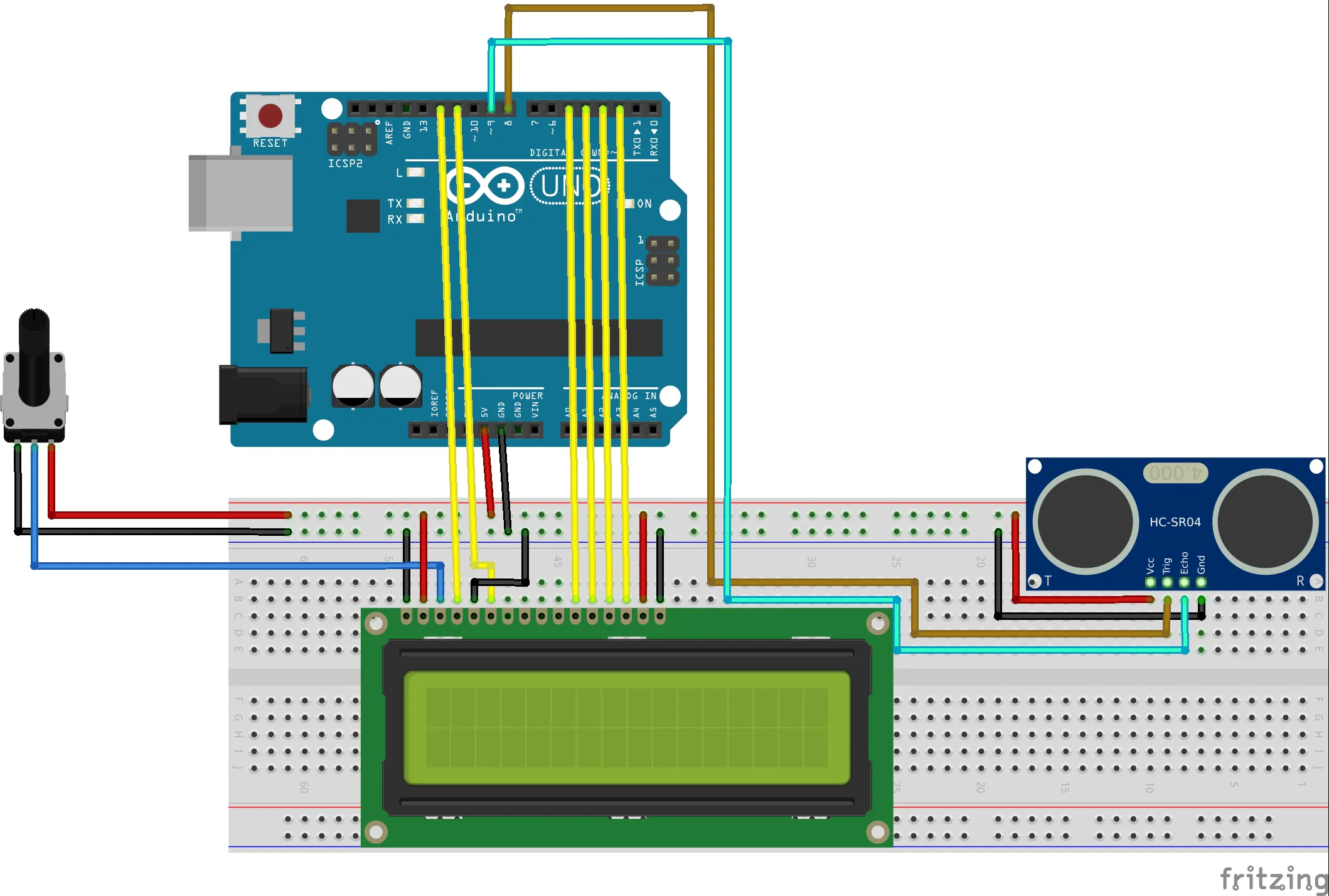### PDDU schematic

PDDU schematic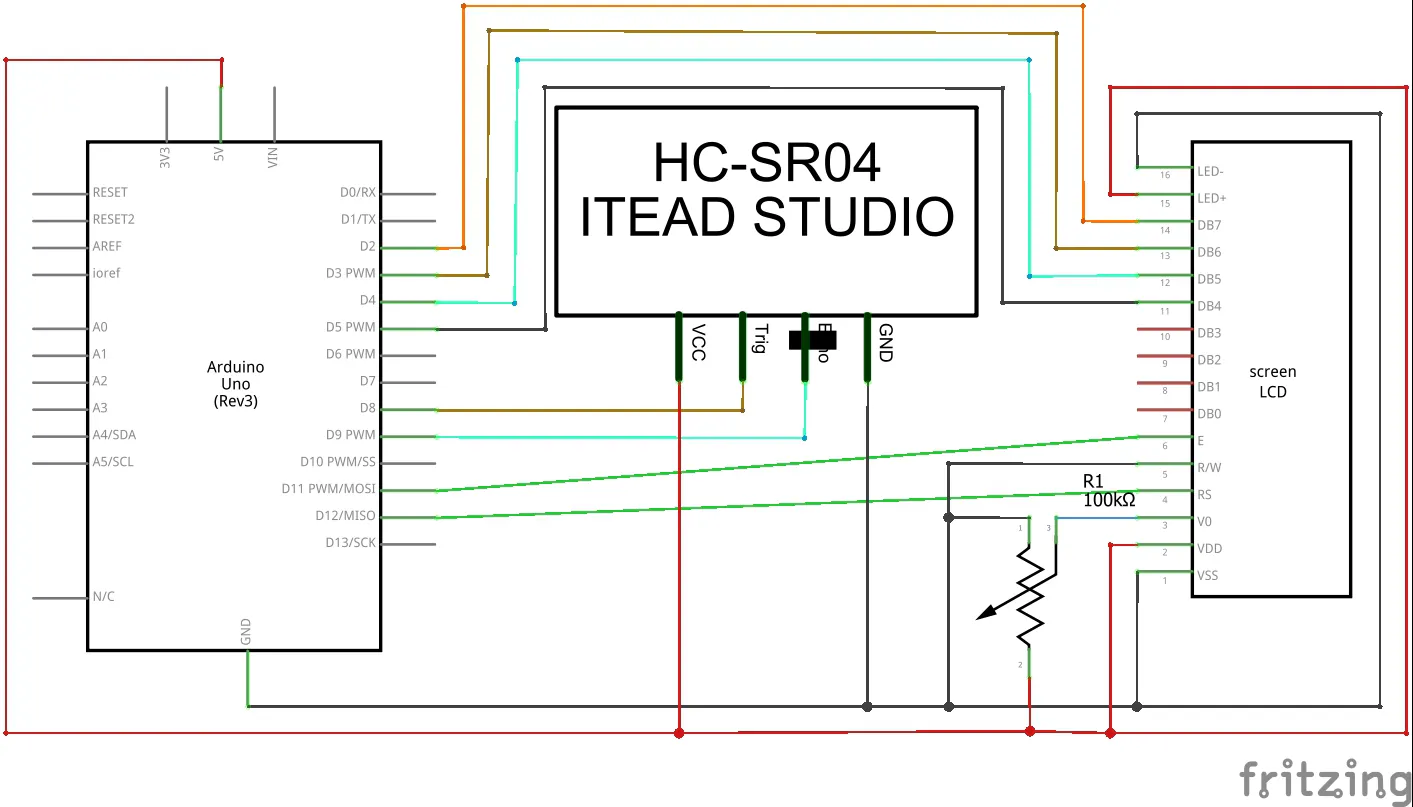## Code

### PDDU code

C/C++
```#include<LiquidCrystal.h>
const int rs=12, en=11, d4=5, d5=4, d6=3, d7=2;
LiquidCrystal lcd(rs, en, d4, d5, d6, d7);
#include<HCSR04.h>
const int Triggerpin=8;
const int EchoPin=9;
long Duration=0;
void setup() {
lcd.begin(16,2);
pinMode(Triggerpin,OUTPUT);
pinMode(EchoPin,INPUT);
}
void loop() {
lcd.noDisplay();
lcd.setCursor(0,0);
digitalWrite(Triggerpin,LOW);
delayMicroseconds(2);
digitalWrite(Triggerpin,HIGH);
delayMicroseconds(10);
digitalWrite(Triggerpin,LOW);
Duration=pulseIn(EchoPin,HIGH);
long Distance_cm=Distance(Duration);
lcd.print("Distance= ");
lcd.setCursor(10,0);
lcd.print(Distance_cm);
lcd.print("cm");
lcd.display();
delay(1000);
lcd.noDisplay();
lcd.setCursor(0,1);
digitalWrite(Triggerpin,LOW);
delayMicroseconds(2);
digitalWrite(Triggerpin,HIGH);
delayMicroseconds(10);
digitalWrite(Triggerpin,LOW);
Duration=pulseIn(EchoPin,HIGH);
long Distancem_m=Distancem(Duration);
lcd.print("Distance= ");
lcd.setCursor(10,1);
lcd.print(Distancem_m);
lcd.print("m");
lcd.display();
delay(3000);
lcd.clear();
}
long Distance(long time){
long DistanceCalc;
DistanceCalc=((time*0.0343)/2);
return DistanceCalc; }
long Distancem(long time){
long DistancemCalc;
DistancemCalc=((time/2915.452)/2);
return DistancemCalc;
}
```